# Low-Noise Amplifier Stability Concept to Practical Considerations, Part 2

### Abstract

Part two of a three-part series. This part covers the RF aspects of low-noise amplifiers. Stability, impedance matching and general amplifier design are covered. Emphasis is on S-parameters as design tools.

In Part 1, we started our discussion with a brief background on transmission lines and a reminder about RF power gain definitions. In this part, we jump into the RF aspect of low noise amplifiers by examining stability (tendency for oscillation), impedance matching, and general amplifier design, using scattering parameters (S-parameters) as design tools.

Part 3 completes the series by presenting application examples. The first shows how to match an LNA in the maximum available gain condition. The second deals with an LNA matched in the constant desired gain condition. The third exercise stresses the importance of matching a potentially unstable LNA in its stable area.

### Stability Calculations

Some say the easiest way to build an oscillator is to design an amplifier. Experience shows this to be true, but it need not be the case. A bit of planning and some basic, a priori knowledge of the LNA to be used can go a long way toward preventing oscillation in an amplifier design.

Two-port networks can be completely characterized by their scattering (S) parameters. Scattering parameters allow the calculation of potential instabilities (trend toward oscillation), maximum available gain, input and output impedances, and transducer gain. S-parameters also allow the calculation of optimum source and load impedances, either for simultaneous conjugate matching or simply to help choose the source and load impedances for a specified transducer gain.

One characteristic of two-port networks is that |S21| ≠ |S12|, and that most of the time |S21| >> |S12|. Consider the following network (an LNA), connected to its source and load impedances. The magnitudes |ΓS| and |ΓL| of the source and load reflection coefficients ΓS and ΓL are less than or equal to 1, which means that the corresponding impedances have positive real parts.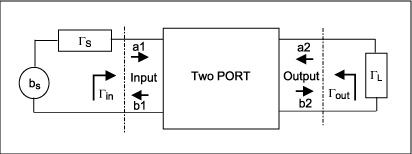First, we study a one-sided two-port network for which S12 = 0. This case is interesting, because it supports a simple example that helps to highlight certain rules without the need for heavy mathematics.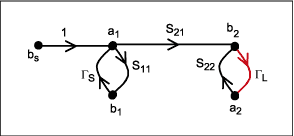In this case we write a simplified version of the transducer gain equation (Eq. 5-5) as:G1 and G2 are called the mismatch gain.

When |S22| < 1 and |S11| < 1, the two-port network is unconditionally stable. As a consequence, the amplifier remains stable for any combination of source and load impedances. Without reverse gain (S12 = 0), the maximum available gain you can hope to achieve with an LNA under the conjugately matched condition (ΓS = S11* and ΓL = S22*) is:These conjugate-matching conditions assume the maximum power transfer from source to load. With no reverse gain (S12 = 0), the source and load impedance have no effect on the device input and output impedance.

When |S22| > 1 or |S11| > 1, the two-port network is potentially unstable, and will oscillate for certain values of source and load impedance ΓS = 1/S11 or ΓL = 1/S22. The conditions |S22| > 1 or |S11| > 1 do not indicate, however, that the network cannot be used as an amplifier. It merely indicates that oscillations may occur if you fail to exercise extreme care in choosing the source and load impedances.

### Two-Port Network Stability in the General Case (S12 Different from Zero)

A two-port network is unconditionally stable if the reflection coefficients Γin and Γout (seen from input and output) have magnitudes < 1, regardless of the loads ΓS ≤ 1 and ΓL ≤ 1 at the two-port network input and output access. Impedances seen from the input and output have positive real parts, and are independent of the load impedances presented at the input and output access.

ΓIN = S11 + (S21ΓLS12 / 1 - S22ΓL) can also be written as ΓIN = (S11 - ΔSΓL/ 1 - S22ΓL) and transformed aswith ΔS = (S11S22 - S21S12).

Reflection coefficient ΓL is a complex value with magnitude |ΓL| ≤ 1. Consequently, it will be located inside a circle C1, centered at the origin (O1) with radius = 1. The location of Γin can be determined by a succession of simple geometrical transformations including multiplication ratios, shiftings, and inversions:When the two-port network output reflection coefficient S22 < 1, the radius of circle C4 is too small (with respect to its center location O4) to surround the center of C1. From the relation between Γin and ΓL, it appears that if ΓL is included inside the circle C1, then Γin is included inside the circle C6 when S22 < 1. The magnitude of the reflection coefficient Γin (radius R6) seen from the two-port network's input is inside the circle C6. Therefore, Γin ≤ 1 if R6 = |S21S12| / |1 - |S22|2| ≤ 1, and then |S21S12| ≤ 1 - |S22|2.

Considering that the radius of circle C1 is 1, we can tell that the two-port network will be unconditionally stable if C6 is totally included inside the circle C1. As a consequence, |O6| + R6 ≤ 1.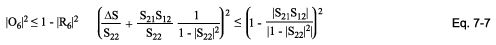O6 and R6 are complex values. To obtain their magnitudes we must square the complex values:

|O6|2 = O6 × O6*.

This last transformation gives the stability factor K, called the Linvill stability factor: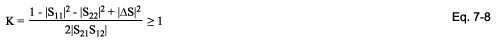Once the condition of unconditional stability has been verified for the reflection coefficient Γin seen from the two-port network input, a similar verification can be made for the output reflection coefficient ΓOUT = S22 + (S21ΓsS12 / 1 - S11Γs) seen from the two port network output. This leads to the results |S21S12| ≤ 1 - |S11|2 and thus Eq. 7-8.

Thus, we have verified that a two port network is unconditionally stable when:

Input : |S21S12| ≤ 1 - |S11|2

Input : |S21S12| ≤ 1 - |S11|2

And the Linvill stability factor (Eq. 7-8) are verified.

Combining the stability conditions on input and output yields the following for a two-port network:

The overall stability conditionWhen K is greater than 1 and ΔS is less than one, the device is unconditionally stable for any combination of source and load impedances. If, on the other hand, K calculates to be less than 1 or ΔS to be greater than 1, the device is potentially unstable and is likely to oscillate for certain combinations of source and load impedance. With K less than 1, or ΔS greater than 1, you must be extremely careful in choosing the source and load impedances. Those conditions do not mean that the device cannot be used, but merely that it will be more difficult to use.

### Potentially Unstable Condition

When the Linvill stability factor indicates potential instability, the device is likely to oscillate with some combination of source and load impedance. When K calculates to be less than 1, therefore, or |ΔS| to be more than 1, it is important to choose the source and load impedances very carefully. One of the best methods for determining which source and load impedances will cause the device to be unstable is to plot stability circles on a Smith chart.

A stability circle is simply a circle on the Smith chart that represents the boundary between those values of source and load impedance that cause instability and those that do not. The perimeter of the circle is the locus of points that force K = 1. Either the inside or the outside of the circle can represent the unstable region, and that determination is made after the circle is drawn. The centers and radii of the input and output stability circles are found as follows.

When a two-port network (LNA) is potentially unstable, we need to identify its stable areas (the values of ΓS and ΓL for which |Γin| ≤ 1 and |Γout| ≤ 1). To define the boundary between stability and instability, we consider the maximum possible value (unity) of the reflection coefficients Γin and Γout seen from the device input and output:For the device output, we demonstrate that the output stability circle C2 (with center O2 and radius R2) is defined by the ΓL values for which |Γin| = 1:The above leads to the equation of a circle in the complex plane: |ΓL – A*|2 = |A|2 - B, centered at A* with a radius of (|A|2 - B)1/2: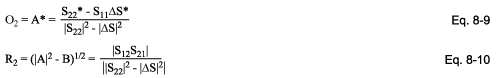The same demonstration can be made for the device input, with ΓS and |Γout|:

Stability circles for device:To determine whether circle C2 surrounds the Smith chart origin, we calculate |O2|2 - R22. If |O2| > R2, the circle does not surround the origin (Figure 8-1). If |O2| < R2, it does: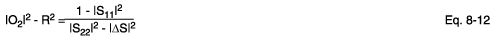If (1 - |S11|2) and (|S22|2 - |ΔS|2) have the same sign (positive or negative), then |O2| is greater than R2, and circle C2 does not surround the Smith chart origin. If (1 - |S11|2) and (|S22|2 - |ΔS|2) have different signs, |O2| is less than R2, and circle C2 does surround the Smith chart origin. After making the stability calculations, you can plot stability circles directly on the Smith chart.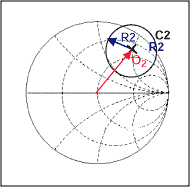For a potentially unstable amplifier, the stability circles might appear as in Figure 8-2 (a-d). As shown, the chart is often intersected by only a portion of the stability circle. After plotting stability circles on the chart, the next step is to determine which side of the circle (inside or outside) represents the stable region.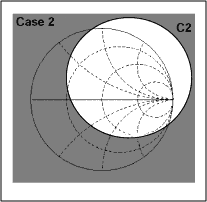Grey areas on the figures represent regions of instability. Choosing ΓL such that ΓIN < 1 ensures that the two-port network is stable at the output. The output conditions in terms of ΓS and ΓIN are also true for the input in terms of ΓL and ΓOUT. To design an oscillator, for example, we might choose ΓL such that ΓIN > 1. That is easily done if S11 and S22 for the device are less than 1.

Because the S parameters were measured with a 50Ω source and load, and because the LNA remained stable for those conditions (S11 and S22 < 1), the center of the normalized Smith chart must be part of the stable region as described by the stability circles. If, therefore, one of the stability circles surrounds the center of the chart in this case, the inside of that circle represents the region of stable impedances for that port.

On the other hand, if S11 or S22 > 1 for an unstable LNA, the circle does not surround the center of the chart. Therefore, the entire area outside that circle represents the stable operating region for the port. Because it is generally wise to do front-end design with the LNA intrinsically stable (S11 < 1 and S22 < 1), only cases 1 and 2 are generally encountered in practical applications. For unconditionally stable amplifiers the entire Smith chart represents a stable operating region (Figure 8-3). Thus, you may never find stability circles to plot for an unconditionally stable LNA.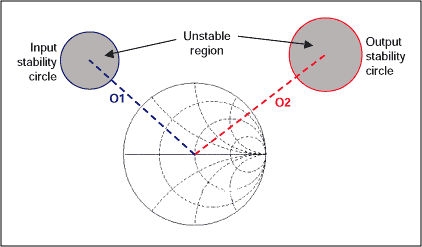### Simultaneous Conjugate Matching (Unconditionally Stable LNA)

For simultaneous conjugate matching of an LNA, it must operate at its maximum available gain. Maximum available gain is the most gain you can expect from a two-port network under the conjugately matched condition.

You can proceed with the design when you find an LNA whose gain capability matches your requirements. The following design procedure yields source and load reflection coefficients that provide a conjugate match for the LNA's actual input and output impedances. Remember that the output impedance of an LNA depends on the source impedance "seen" by the LNA. Conversely, the input impedance of the LNA depends on the load impedance seen by the LNA. These dependencies are caused by the LNA's reverse gain (S12). If S12 is equal to zero, the load and source impedances have no effect on the LNA's input and output impedances.

We now demonstrate how to find the desired load reflection coefficient for a conjugate match, and also how to express the two-port network transducer gain GT = P2 /P1AV as a function of the stability coefficient K. Transducer gain is maximum when power entering the two-port network is maximum (P1 = P1AV) and when power dissipated in the load is maximum (P2 = P2AV). That is, when ΓS = ΓIN* and ΓL = ΓOUT*:By replacing ΓL with its value in the equation for ΓS, we can perform the calculations with the input reflection coefficient ΓS:Next, substitute the calculated value for ΓS (above) in the 2nd-order equation below:Thus,B1 and C1 have to be calculated first, because their values determine which sign (plus or minus) must appear before the radical above. Depending on whether the sign is positive or negative, two solutions ΓS" and ΓS"" are possible for ΓS: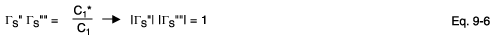Of the two solutions ΓS" or ΓS"", only the one with magnitude < 1 is valid. Making the same calculation with output reflection coefficient ΓL gives a similar result: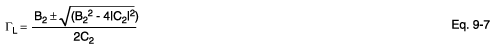With C2 = S22 - S11*ΔS, and B2 = 1 + |S22|2 - |S11|2 - |ΔS|2.

Next, calculate (B12 - 4|C1|2) and (B22 - 4|C2|2) to determine the input and output reflection coefficients (ΓS and ΓL) as a function of the stability factor K. Calculating the input as an example,By replacing the expression 2Real(S11S22*ΔS) in the ΔS function we can write: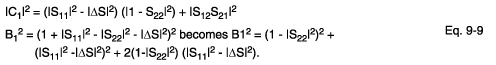Finally,can be expressed as a function of the stability factor K: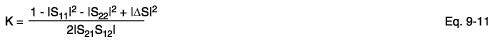As a result, B12 - 4|C1|2 = 4|S12S21|2(K2 – 1).

We can now write ΓS (and ΓL, by changing the index from 1) to 2) in terms of the stability factor K:

For the input:For the output: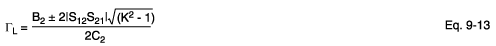To define the sign of ΓS and ΓL, we can use ΓS for the calculations. If the stability factor K > 1, B12 - 4|C1|2 > 0 in this case, and the input reflection coefficient's magnitude (value squared) is:We now determine which sign before the radical (plus or minus) leads to the required condition |ΓS| < 1: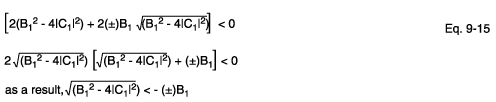As the stability factor K > 1 represents the unconditionally stable condition, we have demonstrated above that:

B1 > 0 → the - sign must be used in ΓS equation for ΓS < 1

B1 < 0 → the + sign must be used in ΓS equation for ΓS < 1

The sign preceding the radical is the opposite of the sign of B1 (previously calculated in Eq. 9-4). The angle of the source reflection coefficient is simply the negative (conjugate) of the angle of the C1 parameter (also found in Eq. 9-4). These relations are also true for calculating the output-matching conditions with B2 > 0 and ΓL.

When the desired source reflection coefficient is found, it can be plotted on a Smith chart, and the corresponding source impedance can be found directly. Or if you prefer, you can substitute ΓS into Eq. 1-2, and solve for ZS mathematically. With the desired source coefficient specified, you can calculate the load-reflection coefficient needed to properly terminate the LNA output (Eq. 9-2):

ΓL = [S22 + (S21ΓSS12 / 1 - S11ΓS)]*

Again, the asterisk indicates that you should take the conjugate of the quantity in parentheses (i.e., same magnitude but opposite sign for the angle). In other words, the magnitude of the result within the parentheses will be correct but the angle will have the wrong sign. Simply change the sign of the angle. Once the desired load reflection coefficient is found, it can be plotted on a Smith chart or substituted into Eq. 1-2 to find the corresponding load impedance.

Next, we verify the conjugate matching condition with stability coefficient K < 1 (the condition indicating a potentially unstable LNA). In that case B12 - 4|C1|2 < 0 (Eq. 9-11). To obtain the roots of this expression, it should be written as an imaginary value: B12 - 4|C1|2 = J2(4|C1|2 - B12).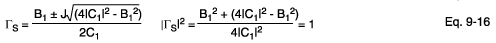With stability coefficient K < 1, the source and load reflection coefficients become equal to 1 when we try to conjugate match the device. As a consequence, we conclude that conjugate input-output matching for an LNA is possible only with an unconditionally stable LNA.

### Gain Definition in Conjugately Matched Conditions: Maximum Available Gain

We have demonstrated that conjugate matching is possible only with the stability coefficient K > 1. In that case (ΓS = ΓIN* and ΓL = ΓOUT*), the transducer gain GT = |S21|2 (1- |ΓS|2) (1 - |ΓL|2 / |(1 - S11ΓS) (1 - ΓOUTΓL)|2 is the same as the available gain:Thenbecomes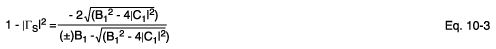The above can be replaced by its value in the transducer gain equation, expressed as:We just have to reintroduce the stability coefficientand it's associated expression (Eq. 9-11): B12 - 4|C1|2 = 4|S12S21|2(K2 – 1).The maximum gain you can obtain from an LNA under conjugately matched conditions is called the maximum available gain (MAG). To define MAG we must first calculate the intermediate quantities B1 and C1 (Eq. 9-4) as done in section 9:

B1= 1 + |S11|2 - |S22|2 - |ΔS|2
C1 = (S11 - S22*ΔS )

B1 should be calculated first, because its polarity determines which sign to use before the radical. For defining ΓS versus stability coefficient K in section 9, we demonstrated that if B1 is positive, the "-" sign must be used, and if B1 is negative the "+" sign must be used. This last rule is also true for the MAG. Note that K must be greater than 1 and ΔS less than 1 (unconditionally stable), or the MAG equation will be undefined. For K > 1 the radical in the equation produces an imaginary number, and the MAG calculation is no longer valid. Thus, the MAG is undefined for unstable LNAs.

For the case K > 1, we express B1 as a function of |C1|2:

|C1|2 = (|S11|2 - |ΔS|2) (|1 - S22|2) + |S12S21|2For stable LNAs, S22 is less than 1. In section 7, where we listed the equation for the stability coefficient K, we stated that |ΔS| < 1. As a consequence, (1 - |S22|2) > |S21S12|. Thus, we can state that B1 > 0 for an unconditionally stable LNA, and therefore the minus sign must be used before the radical. For an unconditionally stable LNA we can express the maximum transducer gain (equal to the maximum available gain) as a function of the stability coefficient.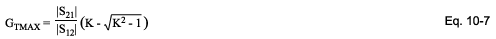The maximum available gain can then be expressed in dB: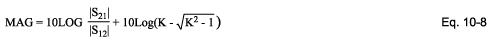For the particular case in which K = 1, GTMAX is defined by GMS = |S21| / |S12|. This is the maximum stable gain.

For the case in which K and ΔS are simultaneously more than 1, the device input and output can be simultaneously matched but it will be potentially unstable. B1 < 1 for this case, so the plus sign must be used before the radical.### Design for a Specified Gain

When designing amplifiers, it is often required that a single stage provide a certain amount of gain—no more, and no less. For that situation, a simultaneous conjugate match for LNA would probably provide too much gain for the stage and would probably overdrive its load (or the succeeding stage). You can search through mountains of literature if you wish, hoping to find an LNA that provides exactly the amount of gain needed when conjugately matched. This approach could take weeks or even months, however. Even if you find an LNA with the gain needed, you are at the mercy of the manufacturer and subject to gain variations among LNAs of the same type. A better way that easily alleviates these problems is called selective mismatching.

Selective mismatching is a controlled and manageable way to limit gain by not matching the LNA to its load. Though it may sound like heresy to some, that technique is supported by a practical, logical, and well-accepted design procedure. Some still believe that an LNA must be matched to its source and load impedances at RF frequencies, but that isn't true. An LNA should be simultaneously conjugate matched to its source and load only if maximum gain is desired, and without regard for other parameters such as noise figure and bandwidth.

One of the easiest methods for selectively mismatching an LNA is through the use of a constant-gain circle plotted on a Smith chart. The circumference of a constant-gain circle represents the locus of points (load impedances) that force the amplifier gain to a specified value. For example, any of the infinite number of impedances located on the circumference of a 10dB constant-gain circle will force the amplifier stage gain to 10dB. When the circle is drawn on a Smith chart, you can see the load impedances that provide a desired gain.

A constant gain circle is plotted on a Smith chart by performing a few calculations to determine the center of the circle and its radius. Constant-gain circles are extrapolated from two-port network power gain (operating or desired). See Eq. 3-9. The following explains how a given power gain equation is written as a circle equation:To simplify the equations, define G = GDESIRED / |S21|2 and D2 = |S22 |2 -|ΔS|2 and C2 = (S22 - ΔSS11*).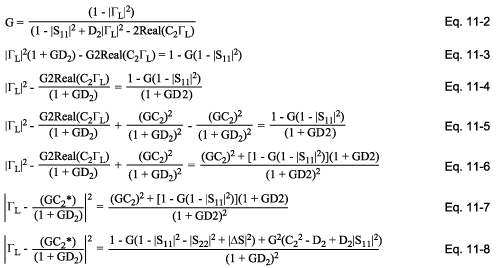The radius for a constant-gain circle can be written as a function of the stability coefficient K. After rearrangement, we have a constant-gain circle equation:The Constant Gain Circles coordinates can be expressed as: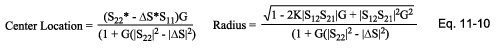Eq. 11-10 produces a complex number for the center location in magnitude-angle format, similar to that of a reflection coefficient. That number is plotted on the chart exactly as you would plot a reflection-coefficient value. The radius of the circle calculated with Eq. 11-10 is simply a fractional number between zero and one, which represents the size of that circle with respect to a Smith chart. A circle of radius "one" has the radius of the Smith chart, a radius of "0.5" represents half the radius of the Smith chart, and so on.

When you choose the load-reflection coefficient (and therefore the load impedance you will use), the next step is to determine the value of source-reflection coefficient needed to complete the design without producing a further decrease in gain. This value is the conjugate of the LNA's actual input reflection coefficient (with specified load), and is given by Eq. 9-2.

In the Smith chart below (Figure 11-1), the circles represent different constant gains (desired gains) that can be obtained with the same LNA. The circle with largest radius represents the lowest constant-gain value. The circle with zero radius represents the maximum available gain condition, in which the LNA input and output are conjugately matched. All circle centers are located on the same axis.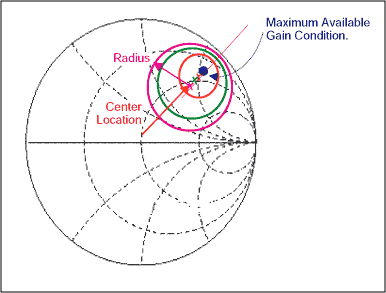### References

Bowick, Chris. RF Circuit Designs. Howard W. Sams & Co. Inc., a publishing subsidiary of ITT.